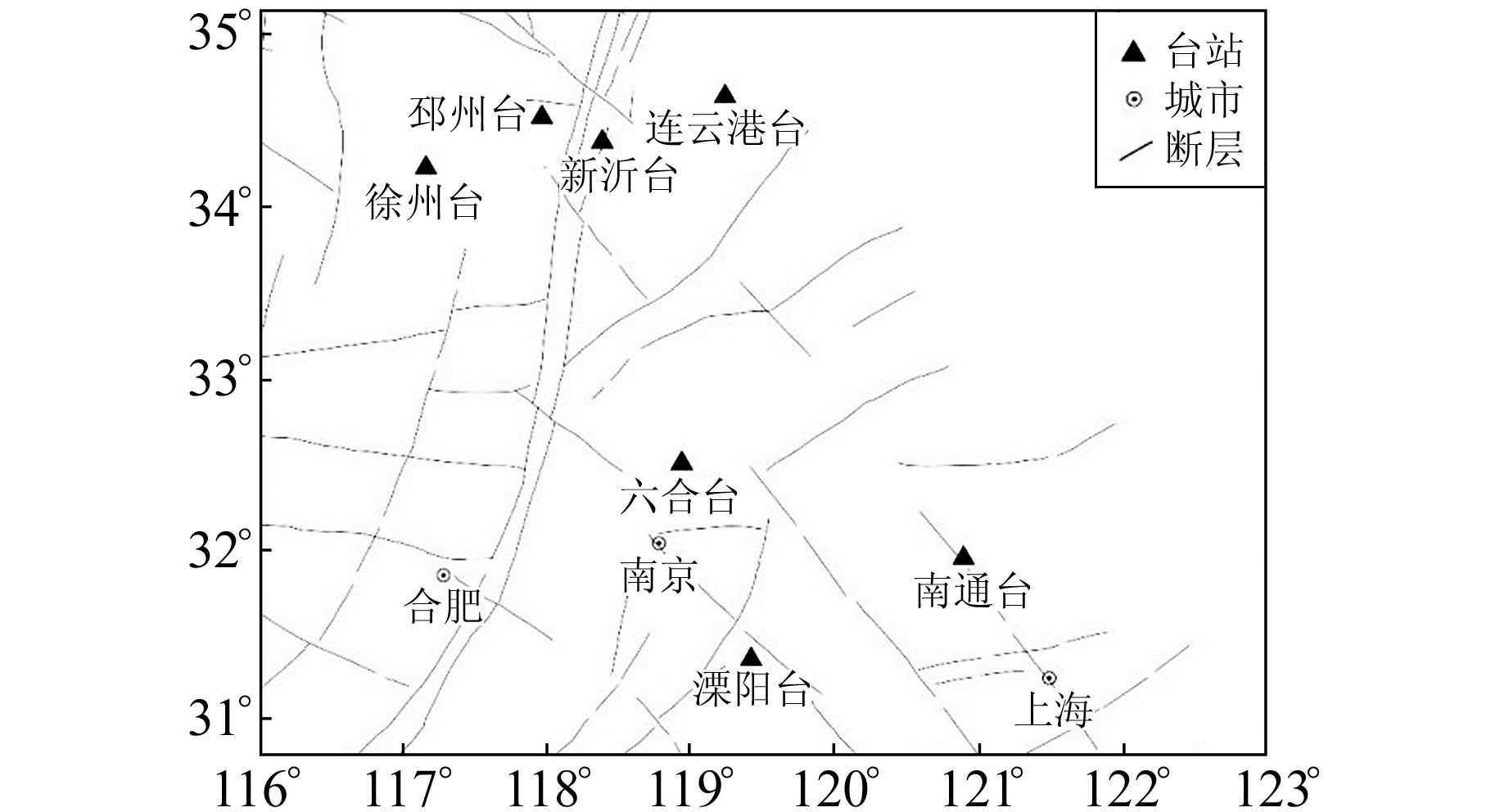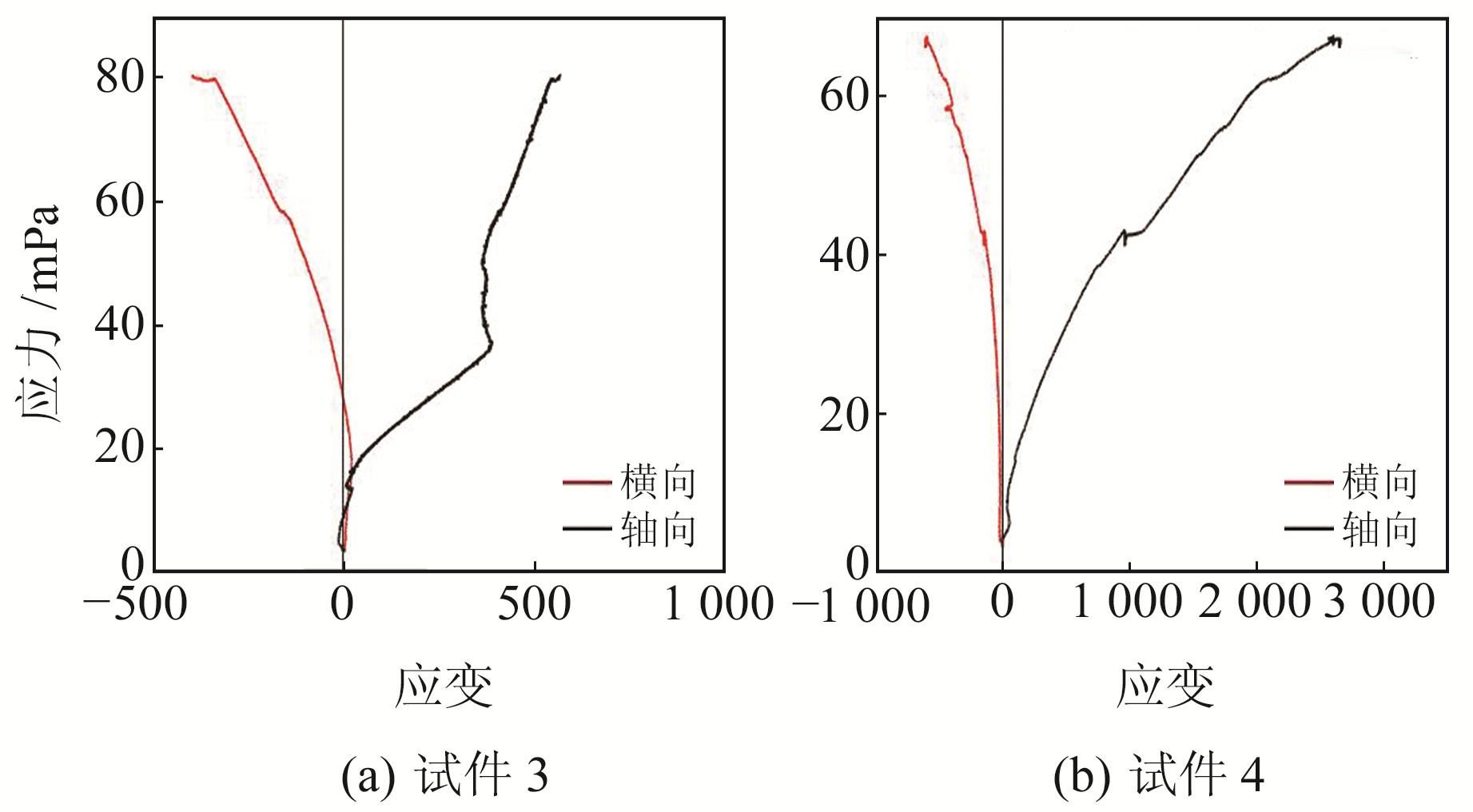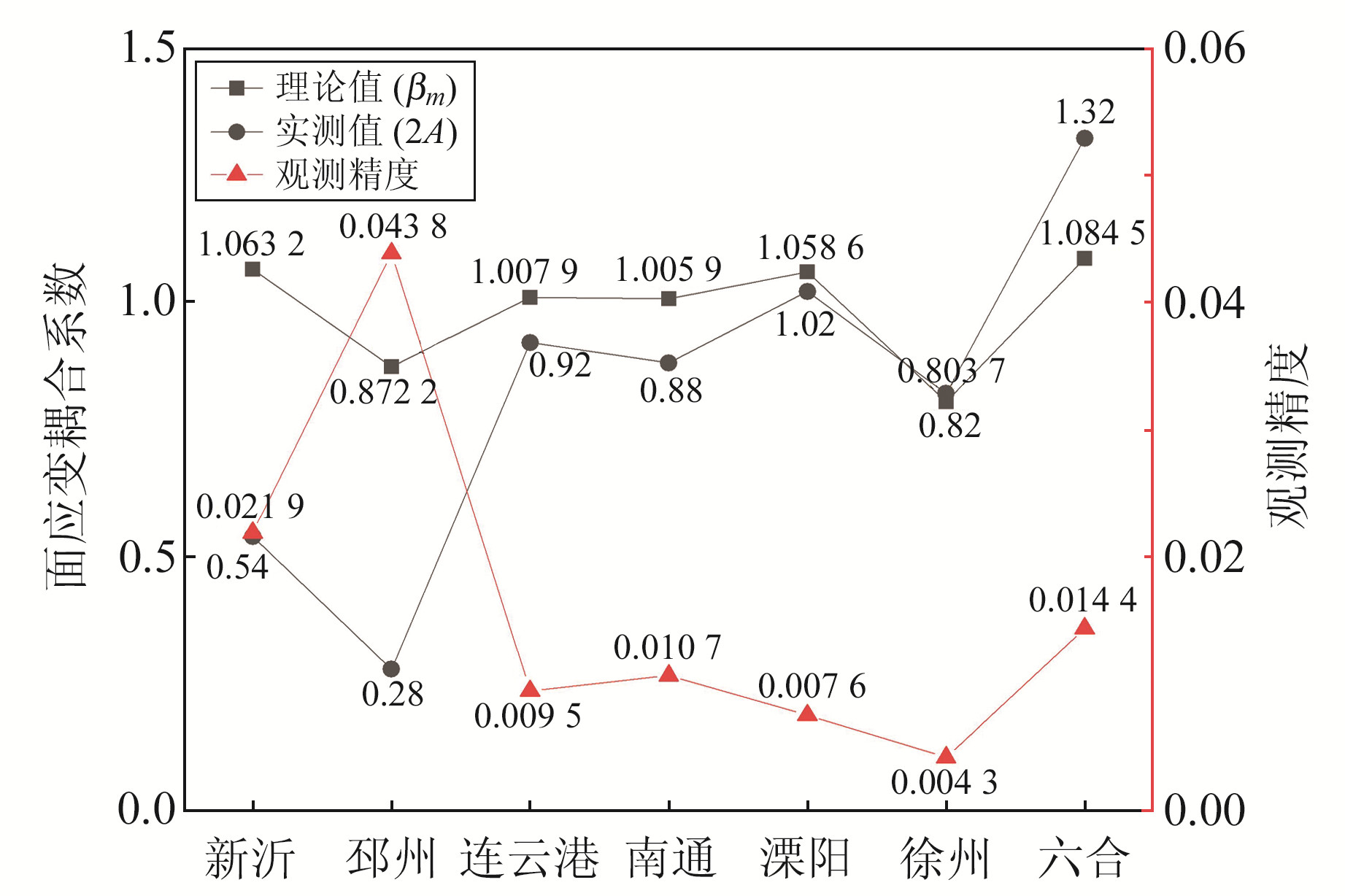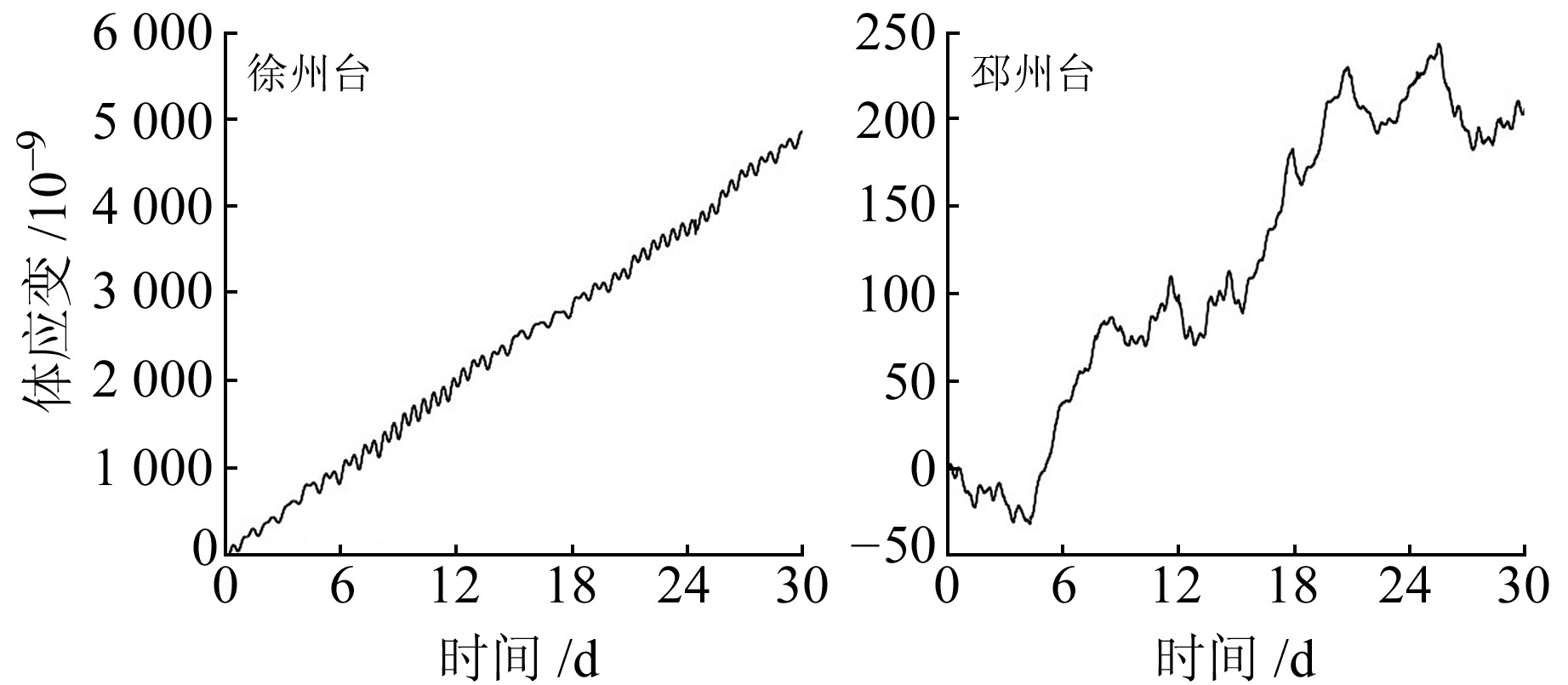﻿ 岩芯力学实验的面应变耦合系数及其差值的阈值研究文章快速检索 高级检索
 大地测量与地球动力学2023, Vol. 43Issue (9): 931-935  DOI: 10.14075/j.jgg.2023.09.010### 引用本文HE Bin, LI Feng, TIAN Tao, et al. Study on the Borehole Coupling Coefficients and the Threshold of Their Difference Base on Core Mechanical Tests[J]. Journal of Geodesy and Geodynamics, 2023, 43(9): 931-935.### Foundation support

The Earthquake Tracking Task of CEA, No.2022010217;Youth Fund of Jiangsu Earthquake Agency, No. 202107.

### 第一作者简介

HE Bin, senior engineer, majors in geophysics and netword deformation observations, E-mail: 85349756@qq.com.

### 文章历史

1. 江苏省地震局，南京市卫岗3号，210014

1 单轴压缩实验 1.1 实验样品与制备图 1 台站分布 Fig. 1 Distribution of the stations表 1 仪器基础信息 Tab. 1 The basic information of the instruments
1.2 实验结果图 2 单轴压缩条件下溧阳台应力-应变曲线 Fig. 2 Stress-strain curves of Liyang station in uniaxial compression test图 3 单轴压缩条件下新沂台应力-应变曲线 Fig. 3 Stress-strain curves of Xinyi station in uniaxial compression test

 $E=\frac{\sigma}{\varepsilon_a}$ (1)

 $\nu=\frac{\varepsilon_b}{\varepsilon_a}$ (2)表 2 各台试件力学参数计算结果 Tab. 2 Calculation results of mechanical parameters of samples at each station

2 面应变耦合系数的计算

2.1 三层复合双衬结构模型

 $\varepsilon_{m i}=\frac{2 k\left(\sigma_1+\sigma_2\right)}{E_3}$ (3)

k值的计算参考张凌空等的双衬套法：

 $k=\frac{E_3 x_4}{E_1}$ (4)
 $x_4=\frac{r_2^2 r_3^2\left(\alpha_2+\beta_2\right)\left(\alpha_3+\beta_3\right)}{r_2^2\left(\alpha_2-\alpha_3\right)\left[r_1^2\left(\alpha_1+\beta_2\right)+r_2^2\left(\beta_1-\beta_2\right)\right]+r_3^2\left(\alpha_3+\beta_3\right)\left[r_1^2\left(\alpha_1-\alpha_2\right)+r_2^2\left(\alpha_2+\beta_2\right)\right]}$ (5)

 $\beta_m=\frac{2 k}{1-\mu_3}$ (6)表 3 各台站的面应变理论耦合系数 Tab. 3 Theoretical coupling coefficient of surface strain at each station
2.2 理论固体潮标定法

 $S=A\left(\varepsilon_x+\varepsilon_y\right)=A\left(\varepsilon_1+\varepsilon_2\right)$ (7)

 $\varepsilon_{\theta \theta}=\frac{1}{R g_0}\left(h_n W_n+l_n \frac{\partial^2 W_n}{\partial \theta^2}\right)$ (8)
 $\varepsilon_{\lambda\lambda}=\frac{1}{R g_0}\left(h_n W_n+l_n \cot \theta \frac{\partial W_n}{\partial \theta}+\frac{l_n}{\sin ^2 \theta} \frac{\partial^2 W_n}{\partial \lambda^2}\right)$ (9)
 $\varepsilon_{\lambda \theta}=\frac{1}{R g_0 \sin \theta}\left(\frac{\partial^2 W_n}{\partial \theta \partial \lambda}-\cot \theta \frac{\partial W_n}{\partial \lambda}\right)$ (10)

 $W_n=W_{m 2}+W_{m 3}+W_{s 2}$ (11)表 4 根据观测值计算的面应变实测耦合系数 Tab. 4 The measured coupling coefficient of surface strain calculated from the observed values

3 面应变耦合系数相关性与观测精度

 $\beta_m=2 A$ (12)图 4 各台站面应变耦合系数与观测精度 Fig. 4 Surface strain coupling coefficient and observation accuracy of each station图 5 徐州台和邳州台2020-03-01~31原始观测曲线 Fig. 5 Original observation curve at Xuzhou and Pizhou stations from March 1 to 31, 2020
4 结语

1) 井下传感器与围岩的耦合程度，是钻孔体应变观测效能的主要影响因素。体应变钻孔岩芯的弹性参数是非常重要的基础数据，通过单轴压缩实验获取相关的弹性模量和泊松比，结合观测模型、系统各组成部分的几何参数获得较为合理的面应变耦合系数。从计算结果来看，江苏区域内整体的面应变理论耦合系数在0.9左右(表 3)。

2) 徐州台、连云港台、南通台和溧阳台的面应变耦合系数理论值和实测值的差值均小于0.1，说明这4个台站的钻孔安装条件和场地条件较好，井下传感器与围岩耦合理想。新沂台和邳州台的面应变耦合系数理论值和实测值的差值均在0.5左右，可能存在井下耦合问题。

3) 钻孔应变仪的观测精度与理论耦合系数和实测耦合系数呈正相关，钻孔应变仪器的理论耦合系数和实测耦合系数一致性越好，仪器的观测精度越高、数据质量越好。江苏省各台站面应变理论耦合系数和实测耦合系数差值的阈值为0.1，当理论耦合系数和实测耦合系数差值小于0.1，说明观测数据质量较高；差值在0.1~0.2之间，说明井下耦合对观测精度有一定影响，但是不显著；当差值超过0.2，说明井下耦合存在问题，仪器的观测精度较低、数据的观测质量较差。

  张凌空, 牛安福. 钻孔体应变与面应变观测井孔耦合系数的计算[J]. 地震学报, 2015, 37(1): 80-88 (Zhang Lingkong, Niu Anfu. Calculation of Borehole Coupling Coefficient Based on Borehole Volume Strain and Area Strain Observation[J]. Acta Seismologica Sinica, 2015, 37(1): 80-88) (0)  张凌空, 牛安福. 基于双衬套理论求解地壳岩石应变传递系数的探讨[J]. 地球物理学进展, 2017, 32(4): 1 525-1 531 (Zhang Lingkong, Niu Anfu. Discussion on Solution of Crustal Rock Strain Transfer Coefficient Based on Double Bush Theory[J]. Progress in Geophysics, 2017, 32(4): 1 525-1 531) (0)  吴庆鹏. 重力学与固体潮[M]. 北京: 地震出版社, 1997 (Wu Qingpeng. Gravity and Solid Tide[M]. Beijing: Seismological Press, 1997) (0)  邱泽华, 石耀霖, 欧阳祖熙. 四分量钻孔应变观测的实地相对标定[J]. 大地测量与地球动力学, 2005, 25(1): 118-122 (Qiu Zehua, Shi Yaolin, Ouyang Zuxi. Relative In-Situ Calibration of 4-Component Borehole Strain Observation[J]. Journal of Geodesy and Geodynamics, 2005, 25(1): 118-122) (0)  何斌, 江昊琳, 章东, 等. 基于单轴压缩实验的钻孔应变仪岩芯力学性质的研究[J]. 地震学报, 2020, 42(6): 697-706 (He Bin, Jiang Haolin, Zhang Dong, et al. Study on the Mechanical Properties of Core about Borehole Strain Meters Based on Uniaxial Compression Experiment[J]. Acta Seismologica Sinica, 2020, 42(6): 697-706) (0)  何斌, 田韬, 王恒知. 基于钻孔特性的体应变观测相关性研究[J]. 地震工程学报, 2018, 40(1): 153-158 (He Bin, Tian Tao, Wang Hengzhi. Study on Correlation of Volume Strain Observation Based on Borehole Features[J]. China Earthquake Engineering Journal, 2018, 40(1): 153-158) (0)  张凌空, 牛安福. 钻孔体应变与面应变观测参数k的计算[J]. 中国地震, 2013, 29(3): 335-346 (Zhang Lingkong, Niu Anfu. Calculation of Borehole Volumetric Strain and Area Strain Observation Parameter k[J]. Earthquake Research in China, 2013, 29(3): 335-346) (0)
Study on the Borehole Coupling Coefficients and the Threshold of Their Difference Base on Core Mechanical Tests
HE Bin1LI Feng1     TIAN Tao1     JIANG HaoLin1     ZHANG Dong1
1. Jiangsu Earthquake Agency, 3 Weigang, Nanjing 210014, China
Abstract: Through uniaxial compression test, we obtain the elastic modulus and Poisson's ratio of the drill core of the borehole strainmeters in Jiangsu province. We calculate the theoretical coupling coefficient and the measured coupling coefficient of the borehole strainmeters using surface strain theory, and discuss their correlation. On this basis, we introduce the observation accuracy, study the relationship between the observation accuracy of the instrument, the coupling coefficient and the data quality, and determine the threshold of the difference of the coupling coefficient. The results show that: 1) The observation accuracy of the borehole strainmeter is positively correlated with the theoretical coupling coefficient and the measured coupling coefficient. The better the consistency between the theoretical coupling coefficient and the measured coupling coefficient, the higher the observation accuracy of the borehole strainmeters and the better the data quality. 2) The threshold of difference between the theoretical coupling coefficient and the measured coupling coefficient of the surface strain at each station in Jiangsu province is 0.1. When the difference is less than 0.1, the observation data quality is higher. When the difference is between 0.1 and 0.2, the downhole coupling has a certain, but not significant, effect on the observation accuracy. When the difference exceeds 0.2, downhole coupling problems occur, resulting in low instrument observation accuracy and poor data observation quality.
Key words: borehole strainmeter; rock mechanics; coupling coefficient; threshold